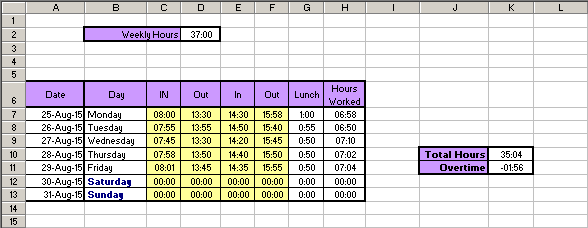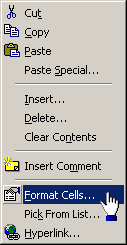# Overtime in Excel: How to calculate itCell Formulae Lunch:   ` =IF(D7="","",IF(E7="","",E7-D7))` Hours worked (for 25th Aug): ` =IF(COUNTBLANK(C7:F7)>0,"",(D7-C7)+(F7-E7))` Total Hours: ` =IF(COUNTBLANK(H7:H11)=5,"",SUM(H7:H11))` Overtime: ` =IF(K10="","",K10-\$D\$2)`Cells containing time references (hours and minutes) should be formatted to "Custom" and "[hh]:mm". To do this all you need to do is select the appropriate cell/s, right-click on a selected cell and choose "Format cells..." from the menu that appears. Select the "Custom" option at the bottom of the list on the left and then enter "[hh]:mm" into the Type box. The [ and ] (that we entered into the Type box) allow the hours value to go outside the 24 hour range.

The '1904 date system' must be set in order to calculate negative hours. To do this use the "Tools" drop-down menu at the top of the window:

```	Tools > Options > Calculation (tab) > 1904 Date System
```
NB: Setting this will mess up any dates already entered onto spreadsheets.